# Chemical Equations and Reactions Types of Chemical Reactions

• Slides: 24Chemical Equations and Reactions Types of Chemical ReactionsTypes of Chemical Reactions Ø 5 basic types l not all reactions fall in these categories Ø you should be able to: l l categorize a reaction by its reactant(s) predict the product(s)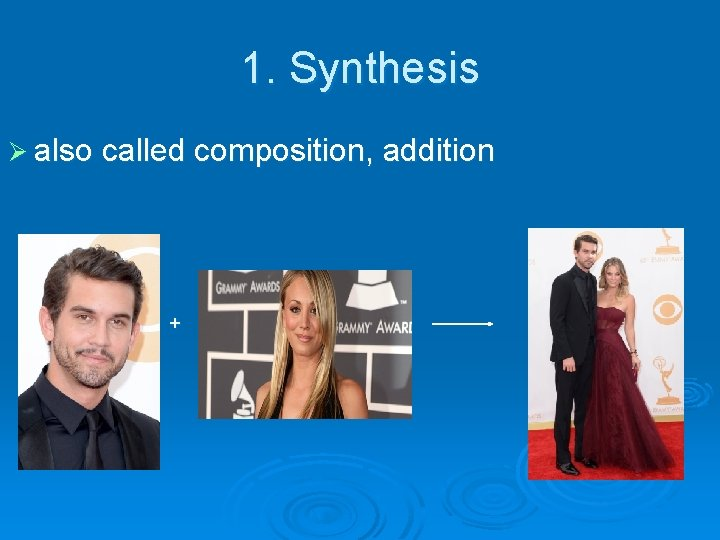1. Synthesis Ø also called composition, addition • +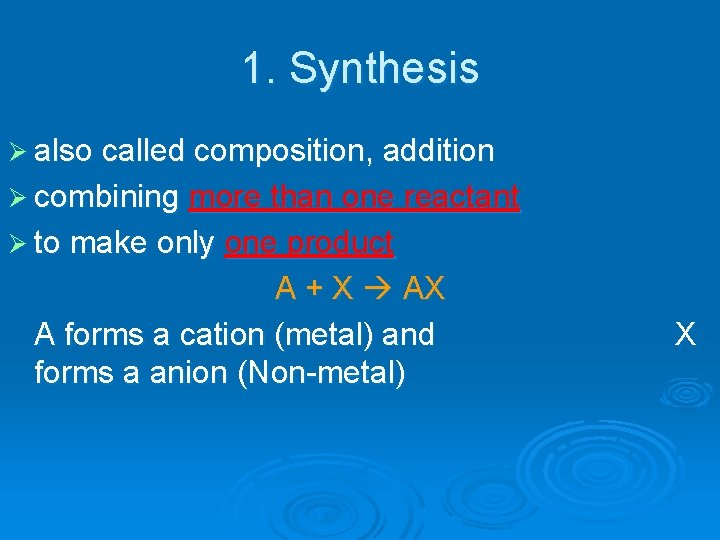1. Synthesis Ø also called composition, addition Ø combining more than one reactant Ø to make only one product A + X AX A forms a cation (metal) and forms a anion (Non-metal) X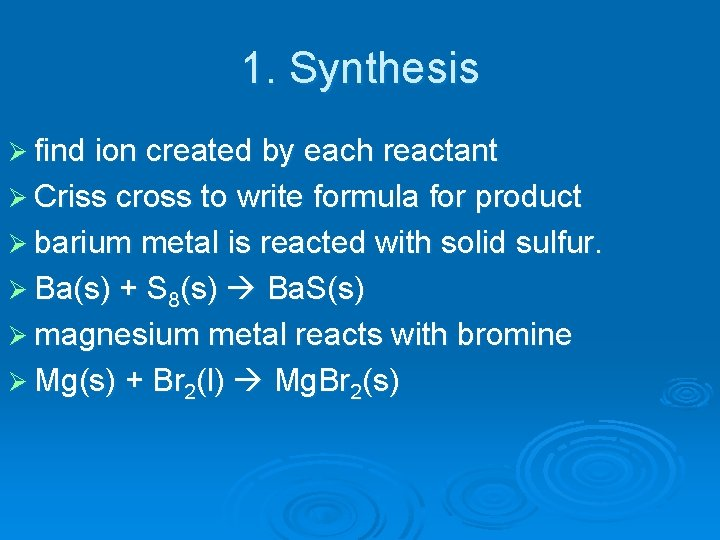1. Synthesis Ø find ion created by each reactant Ø Criss cross to write formula for product Ø barium metal is reacted with solid sulfur. Ø Ba(s) + S 8(s) Ba. S(s) Ø magnesium metal reacts with bromine Ø Mg(s) + Br 2(l) Mg. Br 2(s)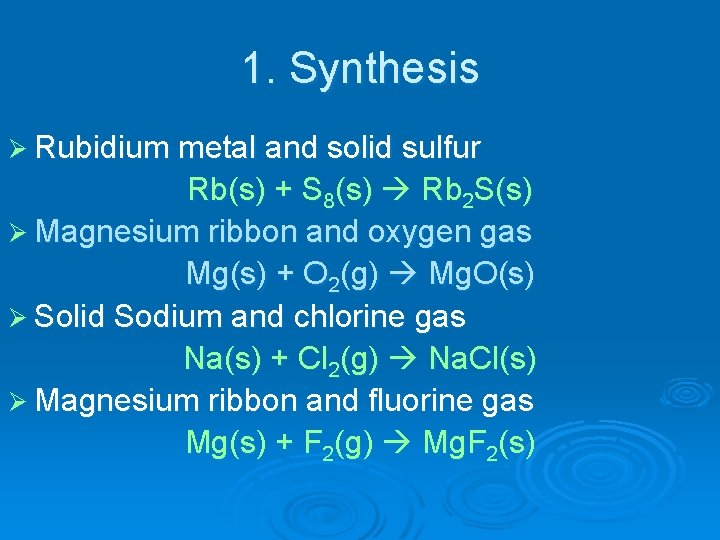1. Synthesis Ø Rubidium metal and solid sulfur Rb(s) + S 8(s) Rb 2 S(s) Ø Magnesium ribbon and oxygen gas Mg(s) + O 2(g) Mg. O(s) Ø Solid Sodium and chlorine gas Na(s) + Cl 2(g) Na. Cl(s) Ø Magnesium ribbon and fluorine gas Mg(s) + F 2(g) Mg. F 2(s)2. Decomposition Ø opposite of synthesis • +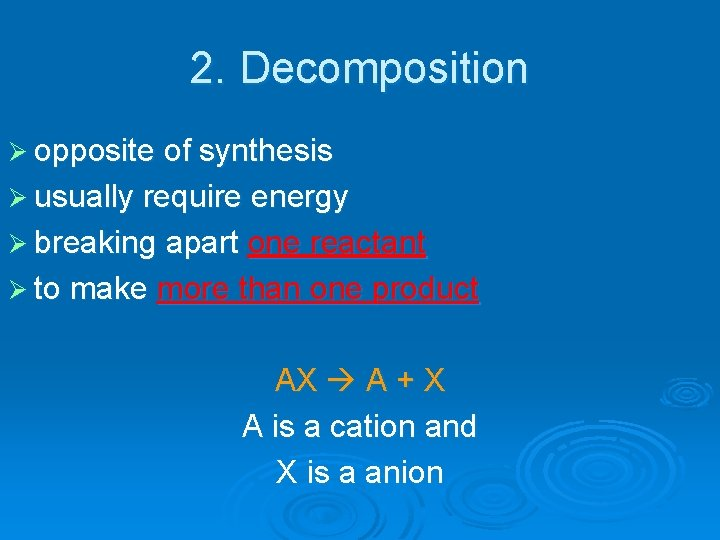2. Decomposition Ø opposite of synthesis Ø usually require energy Ø breaking apart one reactant Ø to make more than one product AX A + X A is a cation and X is a anion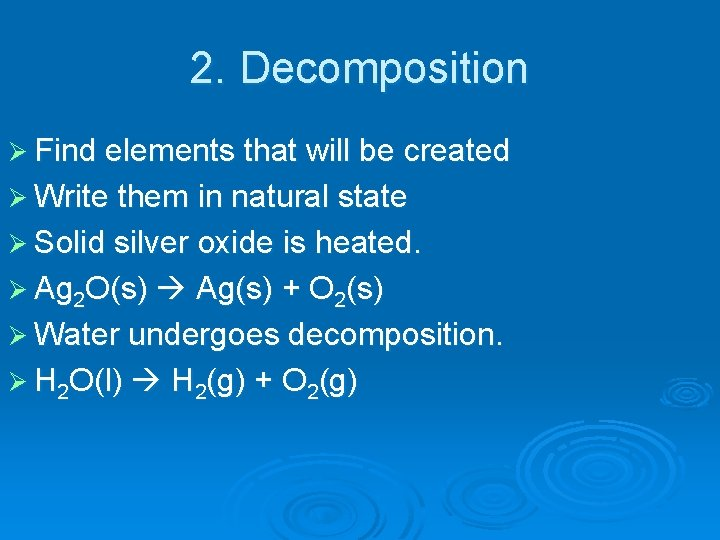2. Decomposition Ø Find elements that will be created Ø Write them in natural state Ø Solid silver oxide is heated. Ø Ag 2 O(s) Ag(s) + O 2(s) Ø Water undergoes decomposition. Ø H 2 O(l) H 2(g) + O 2(g)2. Decomposition Ø liquid water H 2 O(l) H 2(g) + O 2(g) Ø Solid lithium nitride Li 3 N(s) Li(s) + N 2(g) Ø solid aluminum sulfide Al 2 S 3(s) Al(s) + S 8(s)3. Single Replacement Ø an element replaces a similar element in a compound Ø 1 element & 1 compound as reactants Ø 1 element & 1 compound as products Cation (metal) replaced: A + BX B + AX OR anion (nonmetal) replaced: Y + AX X + AY3. Single Replacement + +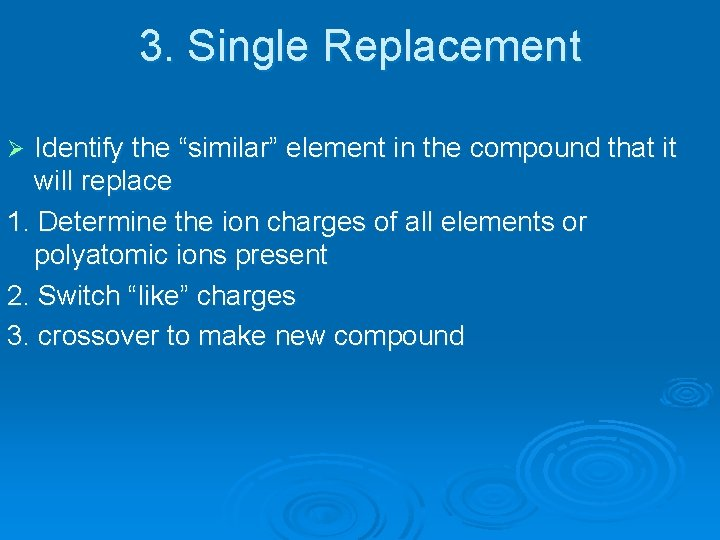3. Single Replacement Identify the “similar” element in the compound that it will replace 1. Determine the ion charges of all elements or polyatomic ions present 2. Switch “like” charges 3. crossover to make new compound Ø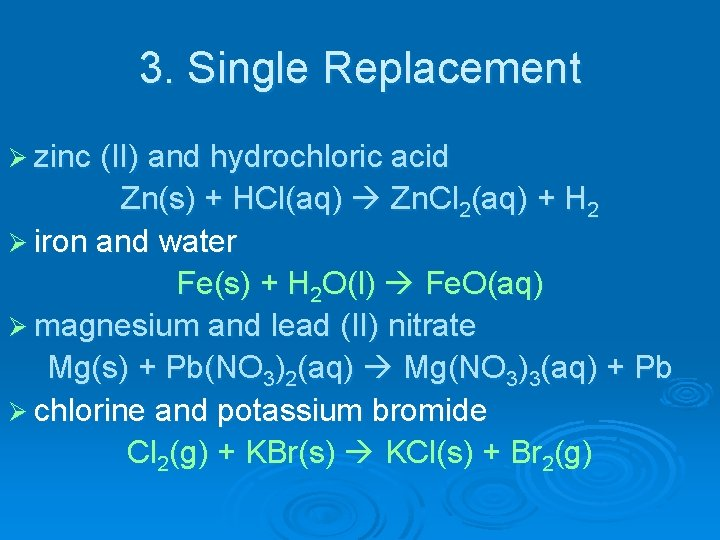3. Single Replacement Ø zinc (II) and hydrochloric acid Zn(s) + HCl(aq) Zn. Cl 2(aq) + H 2 Ø iron and water Fe(s) + H 2 O(l) Fe. O(aq) Ø magnesium and lead (II) nitrate Mg(s) + Pb(NO 3)2(aq) Mg(NO 3)3(aq) + Pb Ø chlorine and potassium bromide Cl 2(g) + KBr(s) KCl(s) + Br 2(g)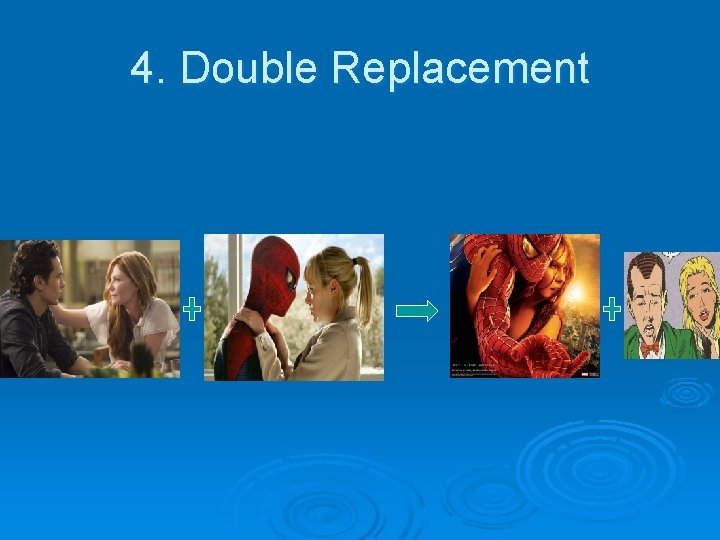4. Double Replacement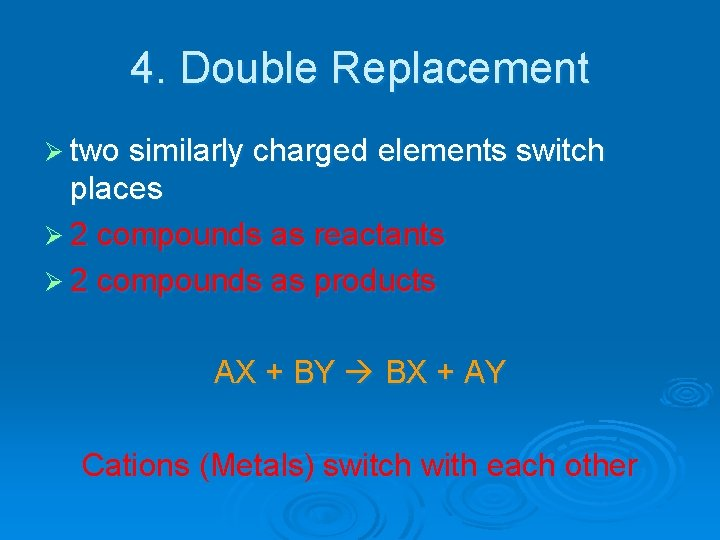4. Double Replacement Ø two similarly charged elements switch places Ø 2 compounds as reactants Ø 2 compounds as products AX + BY BX + AY Cations (Metals) switch with each other4. Double Replacement 1. Identify the cations that will switch places 2. find ions that will be created 3. crossover to create new compounds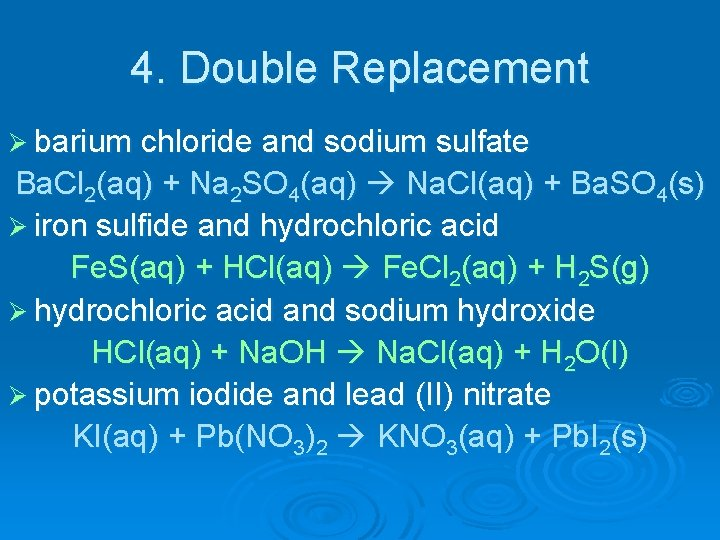4. Double Replacement Ø barium chloride and sodium sulfate Ba. Cl 2(aq) + Na 2 SO 4(aq) Na. Cl(aq) + Ba. SO 4(s) Ø iron sulfide and hydrochloric acid Fe. S(aq) + HCl(aq) Fe. Cl 2(aq) + H 2 S(g) Ø hydrochloric acid and sodium hydroxide HCl(aq) + Na. OH Na. Cl(aq) + H 2 O(l) Ø potassium iodide and lead (II) nitrate KI(aq) + Pb(NO 3)2 KNO 3(aq) + Pb. I 2(s)5. Combustion Ø ALWAYS combines with oxygen, O 2 Ø releases energy in form of heat/light Ø Reactants: Compound (containing H and/or C) + O 2 Ø Products: H 2 O (& usually CO 2) Reactants Products CH 4 + O 2 CO 2 + H 2 O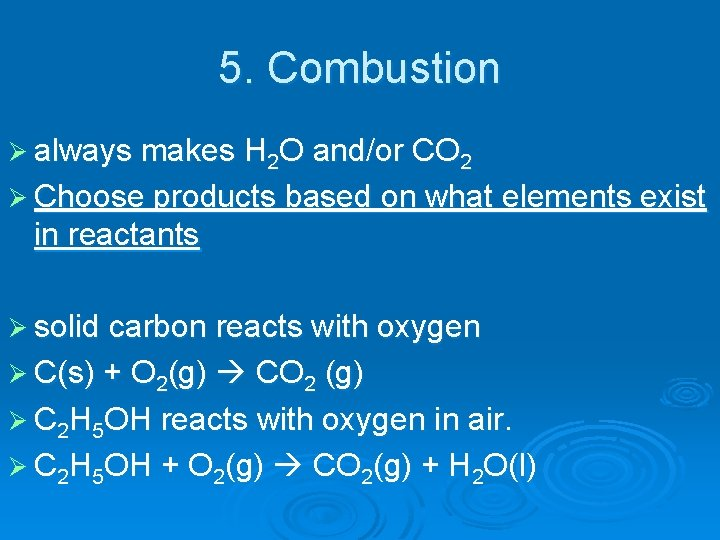5. Combustion Ø always makes H 2 O and/or CO 2 Ø Choose products based on what elements exist in reactants Ø solid carbon reacts with oxygen Ø C(s) + O 2(g) CO 2 (g) Ø C 2 H 5 OH reacts with oxygen in air. Ø C 2 H 5 OH + O 2(g) CO 2(g) + H 2 O(l)Combustion Ø hydrogen and oxygen H 2(g) + O 2(g) H 2 O(g) Ø propane (C 3 H 8) and oxygen C 3 H 8(g) + O 2(g) CO 2(g) + H 2 O(g)Practice Classify each of the following reactions one of the five basic types and predict the products when appropriate. Ø Na 2 O + H 2 O Na. OH l synthesis Ø zinc metal reacts with hydrochloric acid: l l Zn (s) + 2 HCl(aq) Zn. Cl 2(aq) + H 2(g) single replacement Ø Ca(s) + 2 H 2 O(l) Ca(OH)2(aq) + H 2(g) l single replacement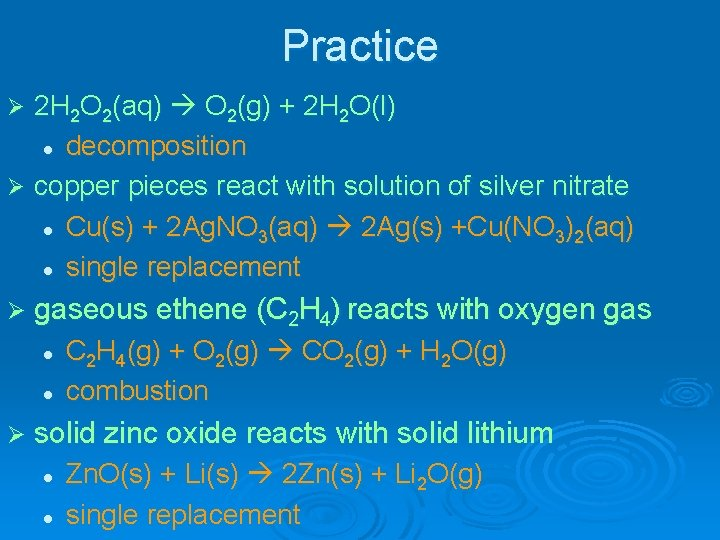Practice 2 H 2 O 2(aq) O 2(g) + 2 H 2 O(l) l decomposition Ø copper pieces react with solution of silver nitrate l Cu(s) + 2 Ag. NO 3(aq) 2 Ag(s) +Cu(NO 3)2(aq) l single replacement Ø Ø gaseous ethene (C 2 H 4) reacts with oxygen gas l l C 2 H 4(g) + O 2(g) CO 2(g) + H 2 O(g) combustion Ø solid zinc oxide reacts with solid lithium l l Zn. O(s) + Li(s) 2 Zn(s) + Li 2 O(g) single replacement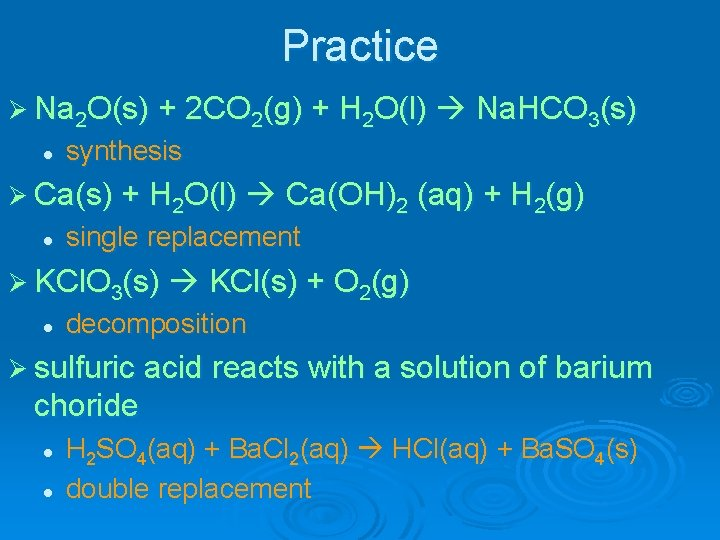Practice Ø Na 2 O(s) + 2 CO 2(g) + H 2 O(l) Na. HCO 3(s) l synthesis Ø Ca(s) + H 2 O(l) Ca(OH)2 l (aq) + H 2(g) single replacement Ø KCl. O 3(s) KCl(s) + O 2(g) l decomposition Ø sulfuric acid reacts with a solution of barium choride l l H 2 SO 4(aq) + Ba. Cl 2(aq) HCl(aq) + Ba. SO 4(s) double replacement